# Plot Run Time Data

Use the Plot tool to track converged and derived solution behavior in real time.

Open the Plot tool in the following ways:
• Select the Plot icon on the Solution ribbon.
• From the Run Status dialog, right-click on an AcuSolve run and select Plot time history from the context menu.
Convergence plots for residual ratios and solution ratios are available by default.

If AcuSolve is still running, the plots update in real time to track the solution progress.

## Create Plots

Select data of interest to plot and then add it to a preview plot. Once all curves are added to the preview plot, the plot is available for placement on the plotting canvas.

1. In the Plot Manager, click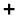to create a new plot.
2. Optional: Click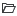to import an existing plot definition.
This allows you to read results data from multiple AcuSolve runs at once and use them as data sources for your plats.
3. Define the y-axis.
1. Select a data type from the Model pane.
Run Data
Contains the general simulation parameters such as Time, Time Increment, CPU Time and Elapsed Time.
Residual ratio
Contains the residual ratios for each of the flow parameters such as pressure, velocity, eddy viscosity and temperature. These ratios can be plotted with three different options Initial, Final and All.
Solution ratio
Contains the solution ratios for each of the flow parameters such as pressure, velocity, eddy viscosity and temperature. These ratios can be plotted with three different options Initial, Final and All.
Surface output
Contains surface integrated values of variables such as pressure, momentum, mass flux and wall shear stress.
Surface statistics
Contains the surface statistics of minimum and maximum of variables such as pressure, momentum, mass flux and wall shear stress.
2. For residual and solution ratios, select a residual type.
Initial
Provides the ratios at the start of each time step.
Final
Provides the ratios at the end of each time step.
All
Provides the ratios for all of the staggers within a time step.
3. For surface output and statistics, select a variable sub-category.
Direction orientated variables, such as velocity, momentum, and shear stress require you to select a coordinate direction.
4. Select the part(s) you want to plot.
4. Optional: Click the x-axis to cycle between values.
5. Optional: Change the name of the plot using the text box below the preview.
7. Select one of the following:
•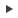- Plot previewed curves and continue creating plots.
•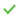- Plot previewed curves and return to the base view.
•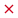- Delete preview and return to the base view.
•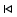- Clear the preview.

## Plot User Defined Functions

Use a python-based scripting language to define new functions from existing curve data.

1. In the Plot Manager, clickto create a new plot.
2. Do one of the following:
• Click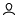.
• Right-click on User Defined in the Model Pane and select Create New.
3. Enter a name for the function.
4. Enter a python script in terms of "Get", "time", "step", or "value".
For example:

cpu = runData(1.'Run Data'.'CPU Time')
elapsed = runData(1.'Run Data'.'Elapsed Time')
value = cpu/elapsed

or

value = 1 + step

5. Select data types, components, and parts to add to the function if needed.
Note: Click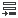to insert the field into the UDF editor with the correct syntax.
6. Select one of the following:
•- Add the function to the plot preview.
•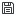- Save the function.
•- Delete the function.
Tip: Click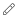to edit a UDF.

## Edit the Plotting Canvas

The canvas layout can be changed to accommodate more plots on a given page. Additional pages can also be created.

1. In the base view of the Plot Manager, clickor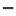to add/delete pages then use the arrows to cycle between them.
2. Use the drop-down on the far right to change the layout of the page and allow for more or less plots.
3. Double-click on an empty plot to select it, then right-click on a data set and select Make Current to plot it.
You can also edit, rename, delete, and export by right-clicking on data sets and variables.
4. Scroll to zoom in or out on a plot and right-click and drag to pan.
Click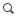to return to a fitted view.
5. To edit display properties, right-click on a curve, plot, or variable.